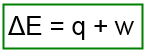# Problem: One mole of H2O(g) at 1.00 atm and 100.°C occupies a volume of 30.6 L. When 1 mole of H2O(g) is condensed to 1 mole of H2O(l) at 1.00 atm and 100.°C, 40.66 kJ of heat is released. If the density of H2O(l) at this temperature and pressure is 0.996 g/cm3, calculate ΔE for the condensation of 1 mole of water at 1.00 atm and 100.°C.

🤓 Based on our data, we think this question is relevant for Professor McCamant's class at UR.

###### FREE Expert Solution

The change in internal energy of a system ΔE is related to heat and work by the equation:Where q is heat and w is work.

The sign of q changes depending on the type of reaction:

(+) q when a system absorbs heat or energy (endothermic)

(-) q when the system releases energy(exothermic)

In the given problem, 40.66 kJ of heat is released when the 1 mole of H2O(g) is condensed to 1 mole of H2O(l). Therefore, the value of q = -40.66 kJ.

Let us now determine the value for work.###### Problem Details

One mole of H2O(g) at 1.00 atm and 100.°C occupies a volume of 30.6 L. When 1 mole of H2O(g) is condensed to 1 mole of H2O(l) at 1.00 atm and 100.°C, 40.66 kJ of heat is released. If the density of H2O(l) at this temperature and pressure is 0.996 g/cm3, calculate ΔE for the condensation of 1 mole of water at 1.00 atm and 100.°C.